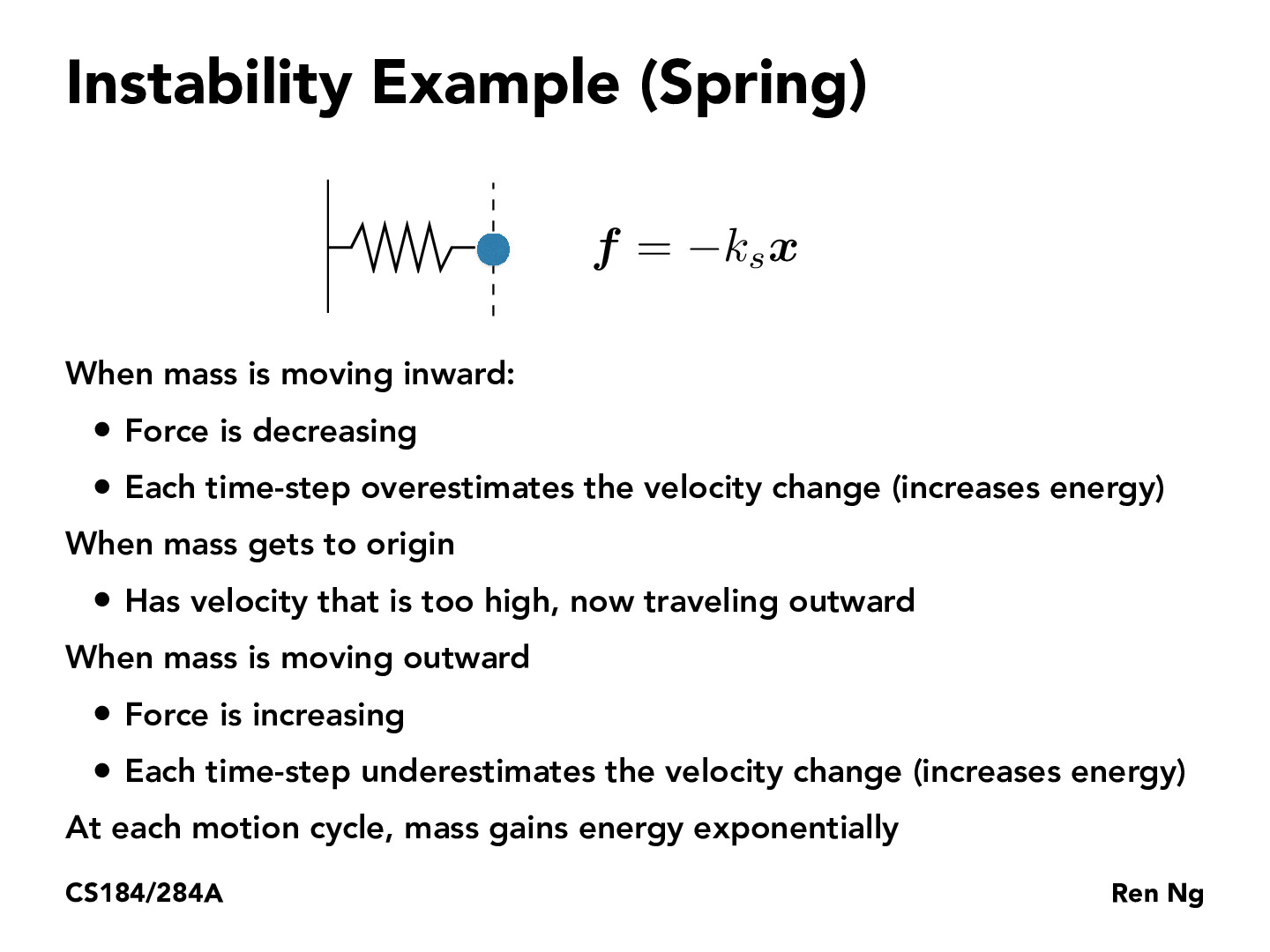You are viewing the course site for a past offering of this course. The current offering may be found here.Lecture 18: Physical Simulation (31)pgujjula

One idea I have to combat instability is to try to maintain invariants such as energy, momentum, and angular momentum, over time. For example, in the spring example, at a given state, we can compute the total potential energy from the spring and the kinetic energy of the ball. We can also compute the total momentum of the system. We may be able to use this extra information to correct the computed velocity and position of the ball over time. For example, we might reduce the velocity of the ball if the computed velocity were too high to satisfy the energy conservation principle or conservation of momentum.archshift

This concept of stability comes from dynamical systems theory. If you haven't taken EE16B, here's a very brief overview of determining whether a given (linear, discrete-time) system is unstable:

First, put your system in the form x[t+1] = A*x[t] + b*u[t] where x is the state of your system and u is the input at a given timestep. A is a matrix.

Then, eigendecompose A.

• If the absolute value of every eigenvalue is < 1, the system is stable.
• If any is == 1, the system is at best marginally stable (any inputs to the system in the dimension of that eigenvalue will destabilize it).
• If any is > 1, the system is unstable.eliot1019

I'm not sure what is going on here, what does it mean for each timestep to overestimate the velocity change?michellebrier

@eliot1019 Forward Euler is estimating an integration over a continuous time interval with discrete time steps, under the assumption that for the entire time interval delta t we have a constant acceleration (the acceleration at the beginning of that time interval). Therefore the problem described in this slide arises, as when the force is decreasing (meaning acceleration is decreasing), this assumption of a constant acceleration causes us to overestimate the velocity change, so we end up with too much velocity when we're back at the origin. The problem continues as we bounce back the other way in our oscillation -- this time we underestimate, so we don't damp as much as we should, which increases energy. And so forth

You must be enrolled in the course to comment